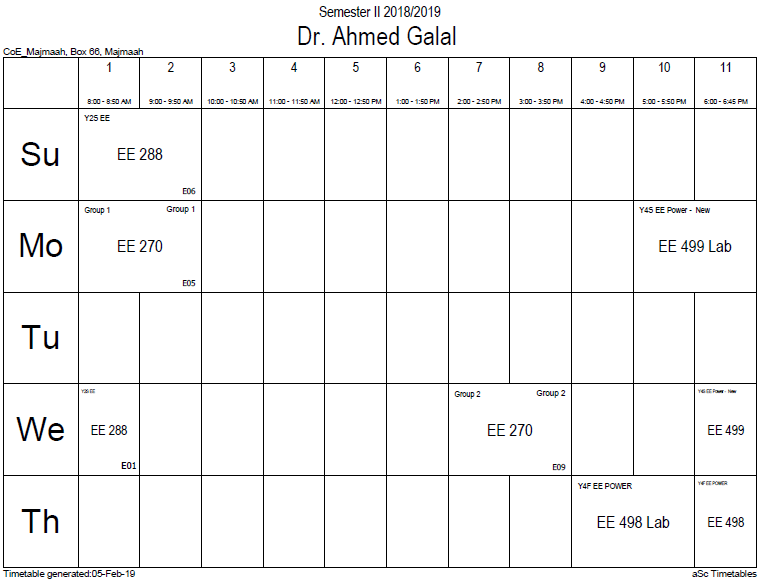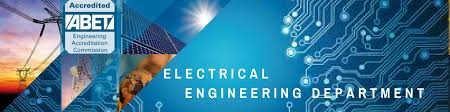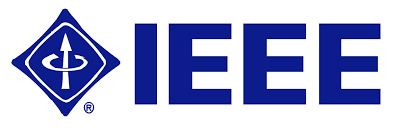## Mathematical logic

Mathematical logic (also known as symbolic logic) is a subfield of mathematics with close connections to the foundations of mathematics, theoretical computer science and philosophical logic. The field includes both the mathematical study of logic and the applications of formal logic to other areas of mathematics. The unifying themes in mathematical logic include the study of the expressive power of formal systems and the deductive power of formal proof systems.

Mathematical logic is often divided into the fields of set theory, model theory, recursion theory, and proof theory. These areas share basic results on logic, particularly first-order logic, and definability. In computer science (particularly in the ACM Classification) mathematical logic encompasses additional topics not detailed in this article; see logic in computer science for those.

Since its inception, mathematical logic has both contributed to, and has been motivated by, the study of foundations of mathematics. This study began in the late 19th century with the development of axiomatic frameworks for geometry, arithmetic, and analysis. In the early 20th century it was shaped by David Hilbert's program to prove the consistency of foundational theories. Results of Kurt Gödel, Gerhard Gentzen, and others provided partial resolution to the program, and clarified the issues involved in proving consistency. Work in set theory showed that almost all ordinary mathematics can be formalized in terms of sets, although there are some theorems that cannot be proven in common axiom systems for set theory. Contemporary work in the foundations of mathematics often focuses on establishing which parts of mathematics can be formalized in particular formal systems, rather than trying to find theories in which all of mathematics can be developed.

### Office HoursNo office hours### My Timetable### Contactsemail: [email protected]

Phone: 2570

### Welcome

Welcome To Faculty of Engineering### IEEEhttp://www.ieee.org/

/

### Bookmarkshttp://www.utk.edu/research/

http://science.doe.gov/grants/index.asp

http://www1.eere.energy.gov/vehiclesandfuels/

http://www.eere.energy.gov/

### Upcoming Conferences### Engineering quotes### Travel Web Siteshttp://www.hotels.com/

http://www.orbitz.com/

http://www.hotwire.com/us/index.jsp

http://www.kayak.com/

### Blackboardستقام اختبارات الميدتيرم يوم الثلاثاء 26-6-1440

حسب الجدول المعلن بلوحات الاعلان

### Summer trainingThe registration for summer training will start from 5th week of second semesterClass registration week 1

### برنامج التجسير### إحصائية الموقع

عدد الصفحات: 2879

البحوث والمحاضرات: 1280

الزيارات: 100897